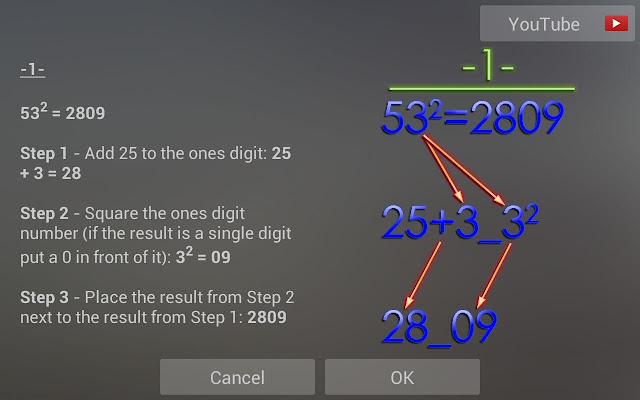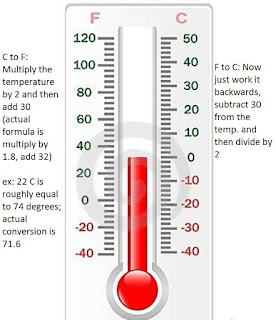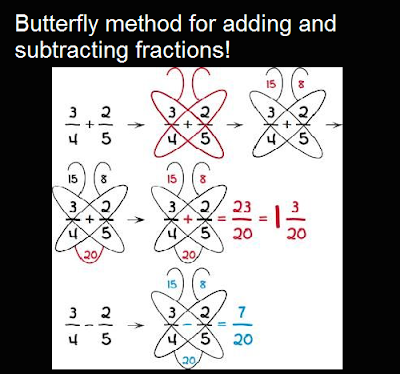### 9 Table:

Just write from 0-9 and 9-0 as shown.Your 9th Table is ready!!

To Multiply two large numbers mentally , just subtract both numbers(here 97 and 96) from 100. Now Add those numbers (here 3 and 4) and subtract from 100 to get first term and mutiply them to get second term.
via

### Square Number:

Follow the simple steps given below.### Fahrenheit Celcius Conversion### Butterfly Method For Fractionsvia

### Easy Mutiplication

Here is a method to reduce your 4 step mutiplication to 2 steps

courtesy:10minuteschool

### Mutiply By Lines

Just Draw lines and jot down the answers easily using this method.

courtesy:10minuteschool

### Multiplying By 11

Any two digit number can be mutliplied by 11 in seconds using this way. Write the two digit as it is and write their sum in between.

via

### Remembering the Value Of Pi

Just Count the letters in the sentence "May I Have a Large Container Of Coffee"
Here

### Guessing The Day

There are few pre set codes for the week days ad months inorder to easily get this job done. Once you  thorough the code, you will do it in seconds.
So Here is the code for Weekdays

Here is the code for Months

Example: April 20 2015
April = 5 (from table)
20 = for dates use 7th table. 7 goes twice, that is,14 = 20 -14 =6
2015= for year find the nearest leap year , that is, 2012 which has a code 1 , that is 1+3 =4
so Monday!!

Another Example
July simply has a code of 5.
20th is 6 because 7 goes into 20 twice, which is 14. 20 – 14 = 6.
2069 is 2 because the leap year code of 2068 is 1 and 2069 is 1 year after, so that’s 2.
The math can be difficult at first because there are a lot of codes, but it works out incredibly well once you pick it up.

One more Example:
January 3, 2014 is a Friday, right?
So, January, according to the table, is 6, and we handle days by using multiples of 7. We don’t need to, so it’s actually just 3 in this case. Next we need to know the closest leap year, which was 2012. That has a year code of 1 and 2013 is 2 years after, so 3 again.
We get 6 + 3 + 3 = 12 – 7 = 5! FRIDAY!

Learn the rules and codes thorough from here